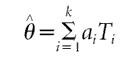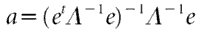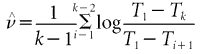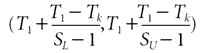## Main

In most cases, the extinction of a species must be inferred from the record of sightings or from collections of individual organisms. But when a species becomes increasingly rare before its final extinction, it may continue to exist unseen for many years — so the time of its last sighting may be a poor estimate of the time of extinction.

We applied an optimal linear estimation method based on the sighting record of the dodo to determine when the bird finally became extinct. Let T1>T2>...>Tk be the k most recent sighting times of a species, ordered from most recent to least recent. Interest centres on using this record to estimate the extinction time, θ. In this context, optimal linear estimation is based on the remarkable result that the joint distribution of the k most recent sighting times has (at least roughly) the same 'Weibull form', regardless of the parent distribution of the complete sighting record3.

Briefly, the optimal linear estimator of θ has the form of a weighted sum of the sighting times, calculated asThe vector of weights is given bywhere e is a vector of k 1's and Λ is the symmetric k × k matrix with typical element λij = (Γ(2^ν + i)Γ(2^ν + j))/(Γ(^ν + i)Γ(j)), ji, and where Γ is the standard gamma function. Also,is an estimate of the shape parameter of the joint Weibull distribution of the k most recent sighting times. An approximate 1 − α confidence interval for θ is given bywhere SL = (−log(1 − α/2)/k)^ν and SU = (−log(α/2)/k)^ν.

The k=10 most recent confirmed sighting times of the dodo are 1662, 1638, 1631, 1628, 1628, 1611, 1607, 1602, 1601 and 1598.

An escaped slave named Simon claimed to have seen a dodo as recently as 1674. However, the reliability of this and other later claims are open to question. For this record, the estimated shape parameter is ^ν = 0.39 and the estimated extinction time is θ̂ = 1690. The approximate 0.95 confidence interval for θ is (1669, 1797). The width of this interval is a result of the low sighting rate of the dodo at the end of its sighting record. Because this rate was so low, it is impossible to rule out an extinction date as late as 1797. This implies that the purported sighting in 1674 cannot be ruled out on the basis of extinction time alone. If a sighting in 1674 is included in the record, the estimated extinction time is extended only modestly to 1700 and actually narrows the confidence interval to (1679, 1790).